Determination of earthquake magnitudes using duration of high-frequency energy radiation and maximum displacement amplitudes: application to the August 31, east of Sulangan, Philippines earthquake

Tatsuhiko Hara

International Institute of Seismology and Earthquake Engineering,

Building Research Institute

We developed a method to determine earthquake magnitudes using the following formula (Hara, 2007a, b):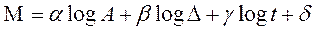where M is an earthquake magnitude,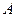is the maximum displacement during high-frequency energy radiation from the arrival time of a P-wave,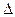is the epicentral distance,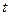is duration of high-frequency energy radiation. The duration of high-frequency energy radiation can be estimated by band-pass filtering of first arriving P-waves (e.g., Hara, 2007a).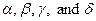are 0.79, 0.83, 0.69, and 6.47, respectively (the units of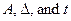were m, km, and s, respectively).

We applied this method to the August 31, east of Sulangan, Philippines earthquake (the origin time: 12:47:34 UTC; the location 10.838°N, 126.704°E after USGS). Figure 1 shows an example of measurements of high-frequency energy radiation. The estimated duration is 30.1 sec. The estimated magnitude using the above formula is 7.8, which is consistent with Mw 7.6 from the Global CMT solution and Mw 7.6 from USGS WPhase Moment Tensor Solution.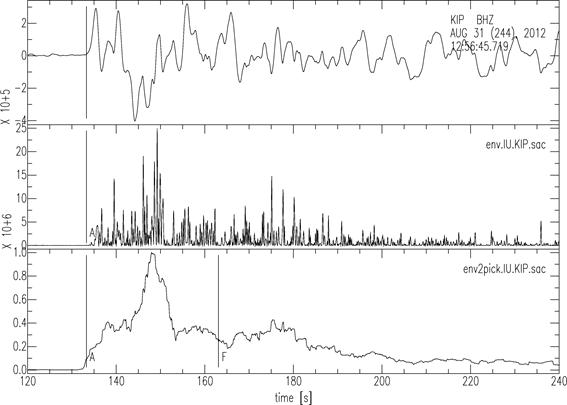Fig. 1. An example of measurements of high frequency energy radiation. The upper, middle and lower traces are an observed seismogram, the squares of the band-pass (2-4 Hz) filtered seismogram, and its smoothed time series (normalized by the maximum value), respectively. “A” and “F” in the lower trace denote the arrival of P-wave and estimated end of high frequency energy radiation, respectively.

References

Hara, T., Measurement of duration of high-frequency energy radiation and its application to determination of magnitudes of large shallow earthquakes, Earth Planets Space, 59, 227–231, 2007a.

Hara, T., Magnitude determination using duration of high frequency energy radiation and displacement amplitude: application to tsunami earthquakes, Earth Planets Space, 59, 561–565, 2007b.

Last Updated: 2012/09/03